Look at Infinite Series | Статья в журнале «Молодой ученый»

Отправьте статью сегодня! Журнал выйдет 1 мая, печатный экземпляр отправим 5 мая.

Опубликовать статью в журнале
Проекты
Меню

Рубрика: Математика

Дата публикации: 09.03.2021

Статья просмотрена: 19 раз

### Библиографическое описание:

Утаганов, Д. О. Look at Infinite Series / Д. О. Утаганов, К. У. Мустаев. — Текст : непосредственный // Молодой ученый. — 2021. — № 11 (353). — С. 4-8. — URL: https://moluch.ru/archive/353/79083/ (дата обращения: 21.04.2021).

﻿

Keywords : infinite series, integral, improper integral, integration by parts, infinitely decreasing geometric progression, differentiate, Riemann zeta function.

In our daily college or school life, we can sometimes face number series as: 1+2+3+…+n. Some of us are able to calculate this kind of number series. What if amount of elements of number series are infinitive? In these cases, it is impossible to calculate value of sum of elements in number series when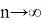. For examples: 1+2+3+…+n is impossible to calculate, however another example is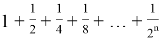, it is obvious that this example can be calculated by using infinite decreasing geometric progression. Our aim is to show a few tough examples of number series which is hard to calculate but possible.

First example: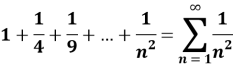Lemma 1: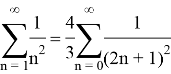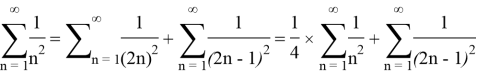Lemma 1 was proved.

Lemma 2: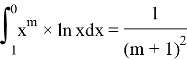To make our life easier we can use integration by parts: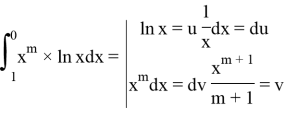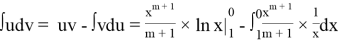=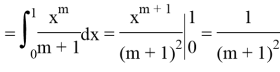Lemma 2 was proved.

We express Lemma 1 in terms of Lemma 2: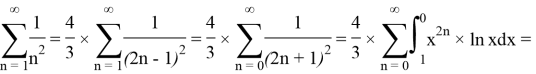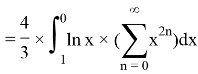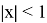because boundaries of integral between 0 and 1 as given. It means that this expression (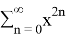) gives us infinitely decreasing geometric progression: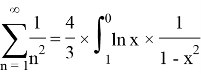We can develop and change our natural logarithms by using limits into: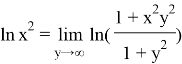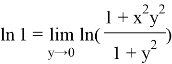We can also rewrite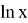as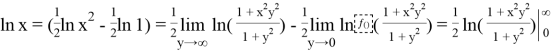Now we have to differentiate this expression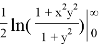with respect to y: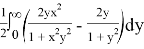It is time to replace natural logarithm to our derived integral above and put all of them to the Lemma 1: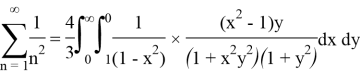It is obvious that we can cancel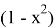, however we will get minus in numerator by canceling, in order to get rid of this minus we should change the boundaries of integral which relates to the X.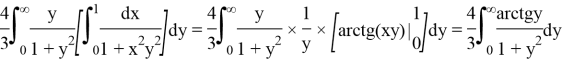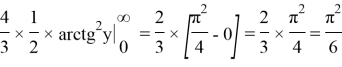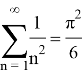Second example: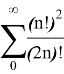Lemma 1: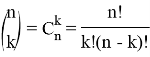By using combinatorics, we can rewrite this expression (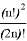) as it shown below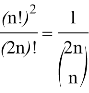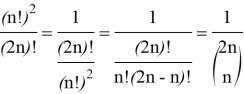Lemma 1 was proved.

Lemma 2: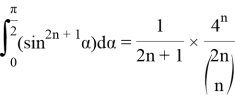Below we will prove mathematical induction: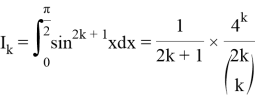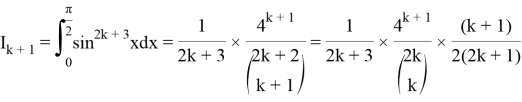To make our life easier we can use integration by parts: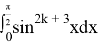=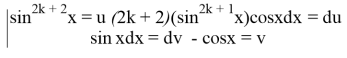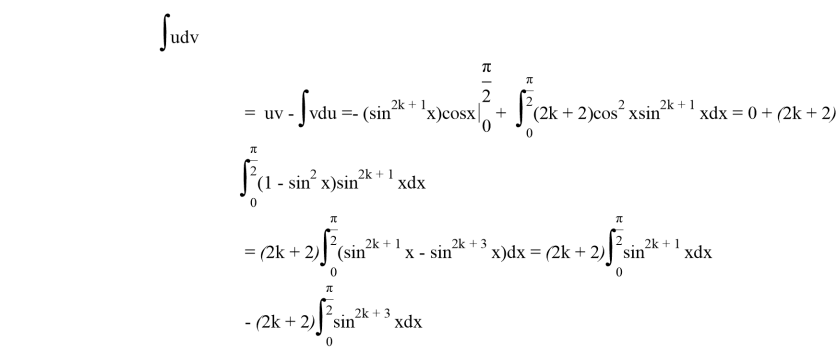Above we can replace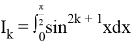and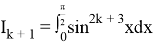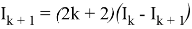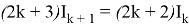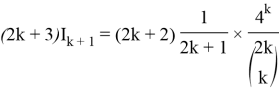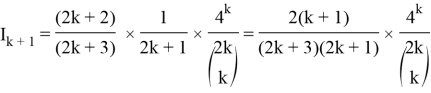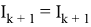which was required to prove.

Lemma 3: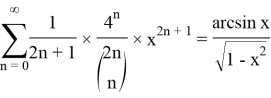We use Lemma 2: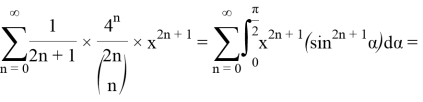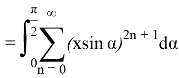At,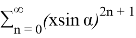we can rewrite as infinitely decreasing geometric progression.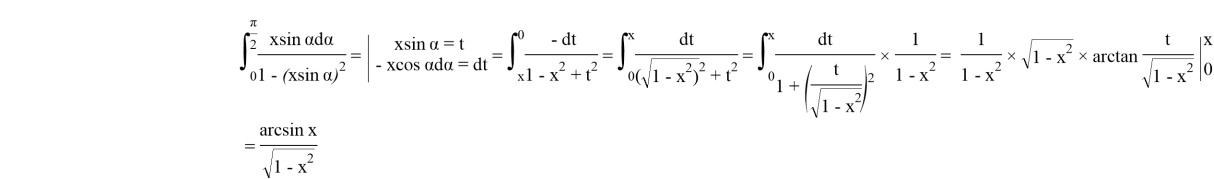Lemma 3 was proved.

We should take a derivative Lemma 3 with respect to X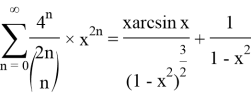As written above at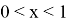so х =, after using Lemma 1 we get an answer of second example: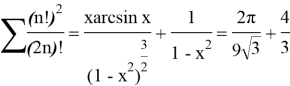Third example: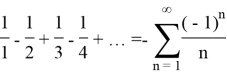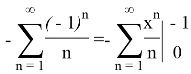Now we have to differentiate this expression with respect to x and write in integral form: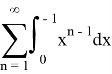because boundaries of integral between 0 and -1 as given. It means that this expression (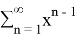) gives us infinitely decreasing geometric progression: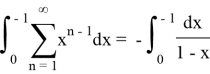After integration we get an answer: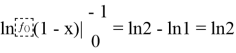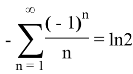Conclusion

Over the examples considered, we wanted to show that the sum of infinite series is not always indefinable, and even if it is possible, it is not easy. For example, we showed that the Riemann Zeta function about n equals 2 is definable, but if n were an odd number, then we could not determine. Finally, we want to say that infinity was and remains a mystery for the world of science.

References:

1. Ter-Krikorov A. M. Mathematical Analysis Course/ A. M. Ter-Krikorov, M. I. Shabunin—6. — Moscow:Binom 2013–672p.
2. Ilyin, V. A. Fundamentals of Mathematical Analysis/ V. A. Ilyin, E. G. Poznyak —7. —Moscow:Fizmatlit, 2001–645p.

## Ключевые слова

integral, infinite series, improper integral, integration by parts, infinitely decreasing geometric progression, differentiate, Riemann zeta function
Задать вопрос